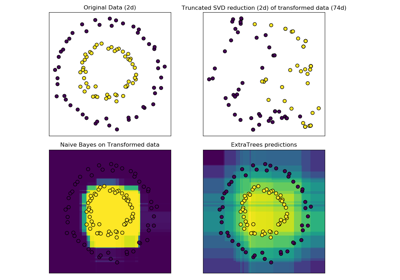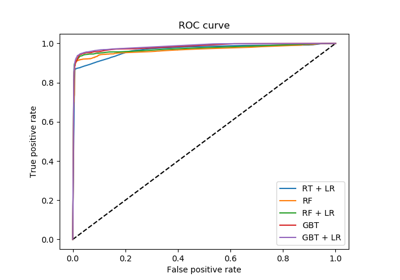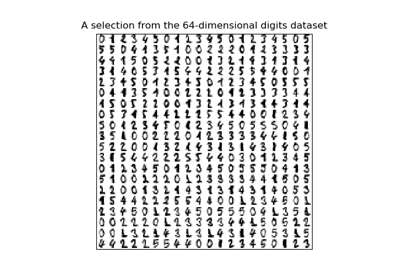# sklearn.ensemble.RandomTreesEmbedding¶

class sklearn.ensemble.RandomTreesEmbedding(n_estimators='warn', max_depth=5, min_samples_split=2, min_samples_leaf=1, min_weight_fraction_leaf=0.0, max_leaf_nodes=None, min_impurity_decrease=0.0, min_impurity_split=None, sparse_output=True, n_jobs=None, random_state=None, verbose=0, warm_start=False)[source]

An ensemble of totally random trees.

An unsupervised transformation of a dataset to a high-dimensional sparse representation. A datapoint is coded according to which leaf of each tree it is sorted into. Using a one-hot encoding of the leaves, this leads to a binary coding with as many ones as there are trees in the forest.

The dimensionality of the resulting representation is n_out <= n_estimators * max_leaf_nodes. If max_leaf_nodes == None, the number of leaf nodes is at most n_estimators * 2 ** max_depth.

Read more in the User Guide.

Parameters: n_estimators : integer, optional (default=10) Number of trees in the forest. Changed in version 0.20: The default value of n_estimators will change from 10 in version 0.20 to 100 in version 0.22. max_depth : integer, optional (default=5) The maximum depth of each tree. If None, then nodes are expanded until all leaves are pure or until all leaves contain less than min_samples_split samples. min_samples_split : int, float, optional (default=2) The minimum number of samples required to split an internal node: If int, then consider min_samples_split as the minimum number. If float, then min_samples_split is a fraction and ceil(min_samples_split * n_samples) is the minimum number of samples for each split. Changed in version 0.18: Added float values for fractions. min_samples_leaf : int, float, optional (default=1) The minimum number of samples required to be at a leaf node. A split point at any depth will only be considered if it leaves at least min_samples_leaf training samples in each of the left and right branches. This may have the effect of smoothing the model, especially in regression. If int, then consider min_samples_leaf as the minimum number. If float, then min_samples_leaf is a fraction and ceil(min_samples_leaf * n_samples) is the minimum number of samples for each node. Changed in version 0.18: Added float values for fractions. min_weight_fraction_leaf : float, optional (default=0.) The minimum weighted fraction of the sum total of weights (of all the input samples) required to be at a leaf node. Samples have equal weight when sample_weight is not provided. max_leaf_nodes : int or None, optional (default=None) Grow trees with max_leaf_nodes in best-first fashion. Best nodes are defined as relative reduction in impurity. If None then unlimited number of leaf nodes. min_impurity_decrease : float, optional (default=0.) A node will be split if this split induces a decrease of the impurity greater than or equal to this value. The weighted impurity decrease equation is the following: N_t / N * (impurity - N_t_R / N_t * right_impurity - N_t_L / N_t * left_impurity)  where N is the total number of samples, N_t is the number of samples at the current node, N_t_L is the number of samples in the left child, and N_t_R is the number of samples in the right child. N, N_t, N_t_R and N_t_L all refer to the weighted sum, if sample_weight is passed. New in version 0.19. min_impurity_split : float, (default=1e-7) Threshold for early stopping in tree growth. A node will split if its impurity is above the threshold, otherwise it is a leaf. Deprecated since version 0.19: min_impurity_split has been deprecated in favor of min_impurity_decrease in 0.19. The default value of min_impurity_split will change from 1e-7 to 0 in 0.23 and it will be removed in 0.25. Use min_impurity_decrease instead. sparse_output : bool, optional (default=True) Whether or not to return a sparse CSR matrix, as default behavior, or to return a dense array compatible with dense pipeline operators. n_jobs : int or None, optional (default=None) The number of jobs to run in parallel for both fit and predict. None means 1 unless in a joblib.parallel_backend context. -1 means using all processors. See Glossary for more details. random_state : int, RandomState instance or None, optional (default=None) If int, random_state is the seed used by the random number generator; If RandomState instance, random_state is the random number generator; If None, the random number generator is the RandomState instance used by np.random. verbose : int, optional (default=0) Controls the verbosity when fitting and predicting. warm_start : bool, optional (default=False) When set to True, reuse the solution of the previous call to fit and add more estimators to the ensemble, otherwise, just fit a whole new forest. See the Glossary. estimators_ : list of DecisionTreeClassifier The collection of fitted sub-estimators.

References

  P. Geurts, D. Ernst., and L. Wehenkel, “Extremely randomized trees”, Machine Learning, 63(1), 3-42, 2006.
  Moosmann, F. and Triggs, B. and Jurie, F. “Fast discriminative visual codebooks using randomized clustering forests” NIPS 2007

Methods

 apply(X) Apply trees in the forest to X, return leaf indices. decision_path(X) Return the decision path in the forest fit(X[, y, sample_weight]) Fit estimator. fit_transform(X[, y, sample_weight]) Fit estimator and transform dataset. get_params([deep]) Get parameters for this estimator. set_params(**params) Set the parameters of this estimator. transform(X) Transform dataset.
__init__(n_estimators='warn', max_depth=5, min_samples_split=2, min_samples_leaf=1, min_weight_fraction_leaf=0.0, max_leaf_nodes=None, min_impurity_decrease=0.0, min_impurity_split=None, sparse_output=True, n_jobs=None, random_state=None, verbose=0, warm_start=False)[source]
apply(X)[source]

Apply trees in the forest to X, return leaf indices.

Parameters: X : array-like or sparse matrix, shape = [n_samples, n_features] The input samples. Internally, its dtype will be converted to dtype=np.float32. If a sparse matrix is provided, it will be converted into a sparse csr_matrix. X_leaves : array_like, shape = [n_samples, n_estimators] For each datapoint x in X and for each tree in the forest, return the index of the leaf x ends up in.
decision_path(X)[source]

Return the decision path in the forest

New in version 0.18.

Parameters: X : array-like or sparse matrix, shape = [n_samples, n_features] The input samples. Internally, its dtype will be converted to dtype=np.float32. If a sparse matrix is provided, it will be converted into a sparse csr_matrix. indicator : sparse csr array, shape = [n_samples, n_nodes] Return a node indicator matrix where non zero elements indicates that the samples goes through the nodes. n_nodes_ptr : array of size (n_estimators + 1, ) The columns from indicator[n_nodes_ptr[i]:n_nodes_ptr[i+1]] gives the indicator value for the i-th estimator.
feature_importances_
Return the feature importances (the higher, the more important the
feature).
Returns: feature_importances_ : array, shape = [n_features]
fit(X, y=None, sample_weight=None)[source]

Fit estimator.

Parameters: X : array-like or sparse matrix, shape=(n_samples, n_features) The input samples. Use dtype=np.float32 for maximum efficiency. Sparse matrices are also supported, use sparse csc_matrix for maximum efficiency. sample_weight : array-like, shape = [n_samples] or None Sample weights. If None, then samples are equally weighted. Splits that would create child nodes with net zero or negative weight are ignored while searching for a split in each node. In the case of classification, splits are also ignored if they would result in any single class carrying a negative weight in either child node. self : object
fit_transform(X, y=None, sample_weight=None)[source]

Fit estimator and transform dataset.

Parameters: X : array-like or sparse matrix, shape=(n_samples, n_features) Input data used to build forests. Use dtype=np.float32 for maximum efficiency. sample_weight : array-like, shape = [n_samples] or None Sample weights. If None, then samples are equally weighted. Splits that would create child nodes with net zero or negative weight are ignored while searching for a split in each node. In the case of classification, splits are also ignored if they would result in any single class carrying a negative weight in either child node. X_transformed : sparse matrix, shape=(n_samples, n_out) Transformed dataset.
get_params(deep=True)[source]

Get parameters for this estimator.

Parameters: deep : boolean, optional If True, will return the parameters for this estimator and contained subobjects that are estimators. params : mapping of string to any Parameter names mapped to their values.
set_params(**params)[source]

Set the parameters of this estimator.

The method works on simple estimators as well as on nested objects (such as pipelines). The latter have parameters of the form <component>__<parameter> so that it’s possible to update each component of a nested object.

Returns: self
transform(X)[source]

Transform dataset.

Parameters: X : array-like or sparse matrix, shape=(n_samples, n_features) Input data to be transformed. Use dtype=np.float32 for maximum efficiency. Sparse matrices are also supported, use sparse csr_matrix for maximum efficiency. X_transformed : sparse matrix, shape=(n_samples, n_out) Transformed dataset.

## Examples using sklearn.ensemble.RandomTreesEmbedding¶Hashing feature transformation using Totally Random TreesFeature transformations with ensembles of treesManifold learning on handwritten digits: Locally Linear Embedding, Isomap…